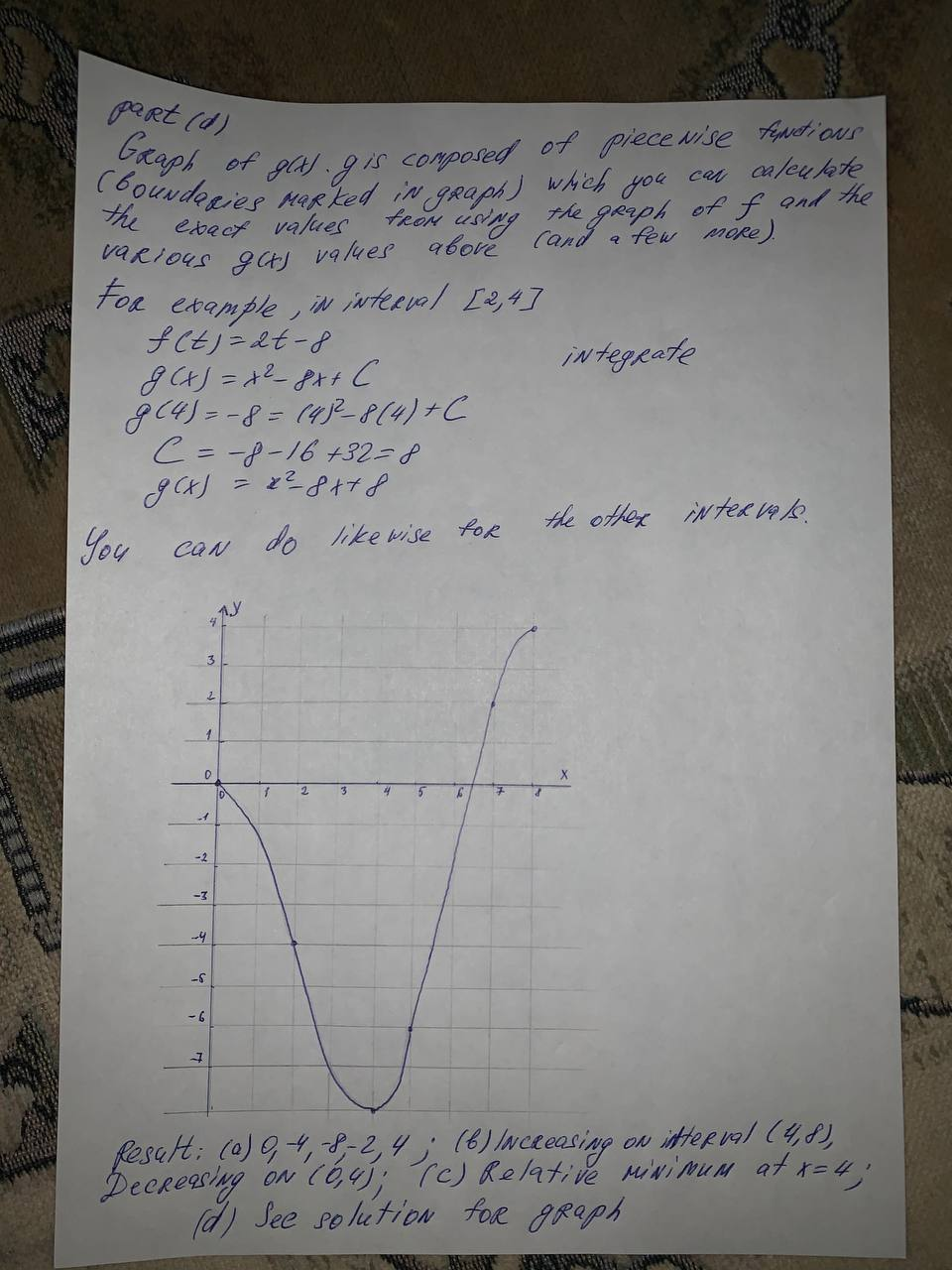#### Didn’t find what you are looking for?

Question# Let g(x) = \int_0^x f(t) dt where f is the function whose graph is shown in the figure. (a) Estimate g(0), g(2), g(4), g(6), and g(8). (b) Find the la

Analyzing functions
ANSWEREDLet $$g(x) = \int_0^x f(t) dt$$ where f is the function whose graph is shown in the figure.
(a) Estimate g(0), g(2), g(4), g(6), and g(8).
(b) Find the largest open interval on which g is increasing. Find the largest open interval on which g is decreasing.
(c) Identify any extrema of g.
(d) Sketch a rough graph of g.